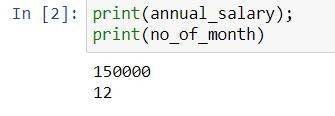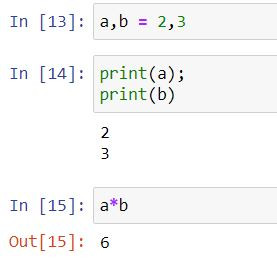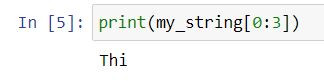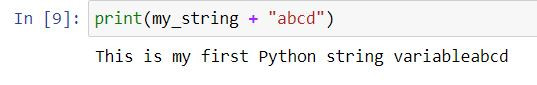Search
•Ekta Aggarwal

# Types of variables in Python

There are majorly 3 types of variables in Python:

Numbers: Can take integers and float (decimal) numbers.

String: Containing alpha numeric and character values.

Boolean : Containing only True and False.

In this tutorial we shall try to understand all of them in detail:

In Python you can assign values to a variable using an equal to ' = ' sign.

## Numbers:

In the following code are assigning a value 150000 to annual_salary variable, while no_of_month is assigned the value equal to 12.

```annual_salary = 150000;
no_of_month = 12;```

You can print the variables to see the values stored in them using print( ) function:

```print(annual_salary);
print(no_of_month)```You can also use these variables to do mathematical computations.

`annual_salary/no_of_month`It is also feasible to save the values of these computations in a variable (So that we can use the computations later on easily).

For instance, we are saving the above calculation in a new variable called monthly_salary.

```monthly_salary = annual_salary/no_of_month
print(monthly_salary)```### type( ) function

We can check the variable type using type( ) function.

We can see that no_of_month is an integer while monthly_salary is a float.

```type(no_of_month)
type(monthly_salary)```### Multiple assignment:

We can assign multiple values to variables in a single statement.

For eg. Below code assigns the value 2 to 'a' and 3 to 'b'.

`a,b = 2,3`## Strings:

String variables can comprise of letters, numbers and special characters eclosed in quotes.

Here we are creating our string:

```my_string = "This is my first Python string variable";
print(my_string)````type(my_string)`Python indexing starts from 0 thus following code will return the first character in the string:

`print(my_string)`Writing [0:3] in front of the string name would provide first 3 characters (i.e. index 0 till index 2, index 3 is excluded)

`print(my_string[0:3])`Following code prints the entire string starting from 3rd index (i.e. 4th character)

`print(my_string[3:])`Writing -1 as the index tells Python to retrieve the last character from our string.

`print(my_string[-1])`### Multiplication with strings:

If we write my_string * 2 then the string will be repeated twice!

`print(my_string * 2)`We can concatenate the strings using a '+' symbol:

`print(my_string + "abcd")`## Boolean:

Here we have calculated a Boolean variable x which takes the value True.

```x = True;
type(x)```Note that in Python while mentioning True and False : T or F is in upper case and rest of the letters in lower case!

Python won't treat TRUE as a Boolean True or FALSE as Boolean False.

Tags:

1 comment

See All